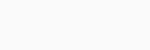Alexa web rank
Trending

# 6 Algorithms Every Developer Should Know

As a developer, it is essential to understand algorithms to create efficient and scalable software applications. Algorithms are sets of instructions that perform a specific task, such as sorting data or searching for information. By learning about these six essential algorithms, you can improve your coding skills and create better applications.

# 6 Algorithms Every Developer Should Know

## Sorting Algorithms:

Sorting algorithms are one of the most common types of algorithms. They are used to arrange data in a specific order, such as ascending or descending order. The most popular sorting algorithms include Bubble Sort, Merge Sort, Quick Sort, and Insertion Sort. Each algorithm has its advantages and disadvantages. For example, Bubble Sort is easy to implement, but it is not efficient for large datasets. In contrast, Merge Sort is efficient for large datasets, but it requires more memory.

## Searching Algorithms:

Searching algorithms are used to find a specific item in a dataset. There are several searching algorithms, including Linear Search, Binary Search, and Jump Search. Linear Search is the simplest searching algorithm, but it is not efficient for large datasets. Binary Search is more efficient than Linear Search, but it requires the dataset to be sorted. Jump Search is a combination of Linear and Binary Search and is efficient for large datasets.

## Graph Algorithms:

Graph algorithms are used to solve problems that involve networks, such as social networks or road maps. The most popular graph algorithms include Breadth-First Search (BFS), Depth-First Search (DFS), and Dijkstra’s Algorithm. BFS and DFS are used to traverse graphs and find all the nodes connected to a particular node. Dijkstra’s Algorithm is used to find the shortest path between two nodes.

## Dynamic Programming:

Dynamic programming is a technique used to solve complex problems by breaking them down into smaller, simpler subproblems. The solutions to these subproblems are then combined to solve the original problem. This technique is used in many algorithms, including the Knapsack Problem, Longest Common Subsequence, and the Fibonacci Series.

## Greedy Algorithms:

Greedy algorithms are used to solve optimization problems. They work by making the best choice at each step, without considering the future consequences. The most popular greedy algorithms include the Minimum Spanning Tree, Dijkstra’s Algorithm, and the Huffman Coding Algorithm. Greedy algorithms are efficient, but they do not always provide the optimal solution.

## Backtracking Algorithms:

Backtracking algorithms are used to solve problems that involve making a sequence of decisions. These algorithms work by exploring all possible solutions and backtracking when a solution is found to be incorrect. The most popular backtracking algorithms include the N-Queens Problem, Sudoku Solver, and Knight’s Tour Problem.

## Conclusion:

These six algorithms are essential for any developer to know. By understanding these algorithms, you can create more efficient and scalable software applications. Keep in mind that these are just a few of the many algorithms out there, and there is always more to learn. Keep exploring and expanding your knowledge of algorithms to become a better developer.

Source

लेख आवडला तर
जास्तीत जास्त 🆂🅷🆁🅴 करा